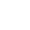Office Closure: Dec 25, 2023, to Jan 1, 2024. Order by Dec 15 for 2023 shipping. Happy holidays from FUTEK!

keyboard_arrow_right

# Guide To Calculating Extraneous Loads

FUTEK Advanced Sensor Technology strongly believes in providing all of our customers with reliable data that helps them in determining the right product for their application. We provide this “Extraneous Loads & Coefficients Guide” in order to help determine the adequacy of the sensor in the presence of loads and moments that are not in the intended direction of the sensor.

Note: In this document all forces, regardless of the load cell’s capacity, are in lb. (pounds mass), and all the moments, regardless of capacity, are in in-lb (inch-pounds).

The forces depicted in Figure 1 may be characterized as following:

Loads (Fx, Fy, Fz [lbs]). Defined as a load along each respective axis, these forces are applied by the customer during or after installation. The direction of each load is indicated on the specification sheet of each load cell model.

Moments (Mx, My, Mz [in-lb]). Moments (torques) are forces that cause the structure to either bend or rotate. To label the axes correctly on each of FUTEK's sensors, please refer to the individual sensor's sheets of each specific model.

Most of the time, the project designer should have a good idea what forces and/or moments will be acting on the sensor. Using this information, FUTEK utilizes an combined stress equation to determine whether or not a sensor is suitable for the application.

There are four easy steps in determining if the external loads are acceptable on the load cell:

2. Determine the extraneous forces and moments that will be acting on the sensor
3. Select the extraneous load coefficients provided by the table for the sensor's capacity
4. Lastly solve the basic equation for the combined stress due to all the loads from step 2. Your calculated combined stress should be equal to or less that the chosen σmax from step 3. If you exceed the allowable stress value, a higher capacity model should be chosen.

The basic equation for combined stress is the following:

σmax ≥ (A)|Fx| + (B)|Fy| + (C)|Fz| + (D)|Mx| + (E)|My| + (F)|Mz|

A, B, C, D, E, F are the coefficients (step 3) determined by FUTEK's engineers. The units of A, B, and C are provided in psi/lbf, whereas the units of D, E, and F are provided in psi/in-lb. The resulting answer from the equation above has units of psi.

## Example

We are looking to see if we can use a 500 lb capacity LCM300 inline load cell for an application. The load cell will experience 300 lb downwards force in the Fz direction, a force of 25 lb in the Fx direction, a 2.5 lb force in the Fy direction, and a moment of 1 in-lb about the z-axis.

To calculate if the combined forces and moments will allow the 500 lb capacity to be used in this application, we need to refer to the External Load Document of the specific load cell.

Table 1: Extraneous Load Coefficient LCM300
CAPACITY (lb) A B C D E F
25 1200 1200 560 3500 3500 1040
50 3500 3500 870 8955 8955 7225
100 3336 3336 530 9050 9050 8345
250 770 770 220 1955 1955 1380
500 665 665 150 1420 1420 1250
1000 475 475 86 1405 1405 1190

We first need to obtain the off axis coefficients (A,B,C,D,E,F) for the desired capacity. We then place the coefficients and forces in the combined stress equation.

Note that the equation requires the absolute value of the forces or moments. For example, although the main force in the Fz direction is applied in the negative direction (-300 lb), the force should be entered as 300 lb in the equation (i.e. |-300|=300).

σ ≥ (A)|Fx| + (B)|Fy| + (C)|Fz| + (D)|Mx| + (E)|My| + (F)|Mz|
σ ≥ 665×|25|+665×|2.5|+150×|-300|+1420×|0|+1420×|0|+1250×|1|
σ ≥ 3325+1662.5+45000+0+0+1250
σ ≥ 64537.5 psi

Next we need to look at the maximum stress (σmax) that the structure is able to handle.

Table 2: Maximum Stresses on the Structure
PARAMETER VALUE σmax
Material 17-4PH S.S.

All the stresses on the structure should be less than or equal to the maximum stress (σmax), depending on the how the load cell is being loaded.

σmax ≥ σ

In our example we see that extraneous loads are acceptable for if they are static or non-reversing. If these loads are fully reversing, the structure might yield, thus a higher capacity unit should be selected. It should be noted that the fully reversing maximum stress condition is determined so that the structure lasts for at least 10 to 20 million cycles. If the load cell needs to last more than 100 million cycles the maximum fatigue stress for a reversing load should be under σmax × 0.75 (46,500 psi in our case). In this case, if we wanted the load cell to last infinite life under fatigue fully reversing conditions, we will need to look for a larger load capacity.Guide-to-calculating-extraneous-loads
All other trademarks, service marks and logos used in this website are the property of their respective owners.guide-to-calculating-extraneous-loads